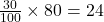## Q. A student earned a grade of 80% on a math test that had 30 problems. How many problems on this test did the student answer correctl

Question

Q. A student earned a grade of 80% on a math test that had 30 problems. How many
problems on this test did the student answer correctly?

in progress 0
2 weeks 2021-09-05T12:31:25+00:00 2 Answers 0 views 0